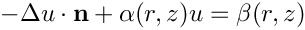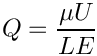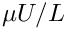oomph::EllipticalTube Class Reference

//////////////////////////////////////////////////////////////////// //////////////////////////////////////////////////////////////////// More...

#include <geom_objects.h>Inheritance diagram for oomph::EllipticalTube:

## Public Member Functions

EllipticalTube (const double &a, const double &b)

EllipticalTube (const EllipticalTube &node)=delete
Broken copy constructor. More...

void operator= (const EllipticalTube &)=delete
Broken assignment operator. More...

double & a ()
Access function to x-half axis. More...

double & b ()
Access function to y-half axis. More...

void position (const Vector< double > &zeta, Vector< double > &r) const
Position vector. More...

void position (const unsigned &t, const Vector< double > &zeta, Vector< double > &r) const

virtual unsigned ngeom_data () const
How many items of Data does the shape of the object depend on? More...

void d2position (const Vector< double > &zeta, RankThreeTensor< double > &ddrdzeta) const
Position Vector and 1st and 2nd derivs w.r.t. zeta. More...

void d2position (const Vector< double > &zeta, Vector< double > &r, DenseMatrix< double > &drdzeta, RankThreeTensor< double > &ddrdzeta) const
Position Vector and 1st and 2nd derivs w.r.t. zeta. More...Public Member Functions inherited from oomph::GeomObject
GeomObject ()
Default constructor. More...

GeomObject (const unsigned &ndim)
Constructor: Pass dimension of geometric object (# of Eulerian coords = # of Lagrangian coords; no time history available/needed) More...

GeomObject (const unsigned &nlagrangian, const unsigned &ndim)
Constructor: pass # of Eulerian and Lagrangian coordinates. No time history available/needed. More...

GeomObject (const unsigned &nlagrangian, const unsigned &ndim, TimeStepper *time_stepper_pt)
Constructor: pass # of Eulerian and Lagrangian coordinates and pointer to time-stepper which is used to handle the position at previous timesteps and allows the evaluation of veloc/acceleration etc. in cases where the GeomData varies with time. More...

GeomObject (const GeomObject &dummy)=delete
Broken copy constructor. More...

void operator= (const GeomObject &)=delete
Broken assignment operator. More...

virtual ~GeomObject ()
(Empty) destructor More...

unsigned nlagrangian () const
Access function to # of Lagrangian coordinates. More...

unsigned ndim () const
Access function to # of Eulerian coordinates. More...

void set_nlagrangian_and_ndim (const unsigned &n_lagrangian, const unsigned &n_dim)
Set # of Lagrangian and Eulerian coordinates. More...

TimeStepper *& time_stepper_pt ()
Access function for pointer to time stepper: Null if object is not time-dependent. More...

TimeSteppertime_stepper_pt () const
Access function for pointer to time stepper: Null if object is not time-dependent. Const version. More...

virtual Datageom_data_pt (const unsigned &j)
Return pointer to the j-th Data item that the object's shape depends on. This is implemented as a broken virtual function. You must overload this for GeomObjects that contain geometric Data, i.e. GeomObjects whose shape depends on Data that may contain unknowns in the overall Problem. More...

virtual void position (const double &t, const Vector< double > &zeta, Vector< double > &r) const
Parametrised position on object: r(zeta). Evaluated at the continuous time value, t. More...

virtual void dposition_dt (const Vector< double > &zeta, const unsigned &j, Vector< double > &drdt)
j-th time-derivative on object at current time:. More...

virtual void dposition (const Vector< double > &zeta, DenseMatrix< double > &drdzeta) const
Derivative of position Vector w.r.t. to coordinates:= drdzeta(alpha,i). Evaluated at current time. More...

virtual void locate_zeta (const Vector< double > &zeta, GeomObject *&sub_geom_object_pt, Vector< double > &s, const bool &use_coordinate_as_initial_guess=false)
A geometric object may be composed of may sub-objects (e.g. a finite-element representation of a boundary). In order to implement sparse update functions, it is necessary to know the sub-object and local coordinate within that sub-object at a given intrinsic coordinate, zeta. Note that only one sub-object can "cover" any given intrinsic position. If the position is at an "interface" between sub-objects, either one can be returned. The default implementation merely returns, the pointer to the "entire" GeomObject and the coordinate, zeta The optional boolean flag only applies if a Newton method is used to find the value of zeta, and if true the value of the coordinate s is used as the initial guess for the method. If the flag is false (the default) a value of s=0 is used as the initial guess. More...

virtual void interpolated_zeta (const Vector< double > &s, Vector< double > &zeta) const
A geometric object may be composed of many sub-objects each with their own local coordinate. This function returns the "global" intrinsic coordinate zeta (within the compound object), at a given local coordinate s (i.e. the intrinsic coordinate of the sub-GeomObject. In simple (non-compound) GeomObjects, the local intrinsic coordinate is the global intrinsic coordinate and so the function merely returns s. To make it less likely that the default implementation is called in error (because it is not overloaded in a derived GeomObject where the default is not appropriate, we do at least check that s and zeta have the same size if called in PARANOID mode. More...

## Private Attributes

double A
x-half axis More...

double B
x-half axis More...Protected Attributes inherited from oomph::GeomObject
unsigned NLagrangian
Number of Lagrangian (intrinsic) coordinates. More...

unsigned Ndim
Number of Eulerian coordinates. More...

TimeStepperGeom_object_time_stepper_pt

## Detailed Description

//////////////////////////////////////////////////////////////////// ////////////////////////////////////////////////////////////////////

Elliptical tube with half axes a and b.Definition at line 1130 of file geom_objects.h.

## ◆ EllipticalTube() [1/2]

 oomph::EllipticalTube::EllipticalTube ( const double & a, const double & b )
inline

Definition at line 1134 of file geom_objects.h.

## ◆ EllipticalTube() [2/2]

 oomph::EllipticalTube::EllipticalTube ( const EllipticalTube & node )
delete

Broken copy constructor.

## ◆ a()

 double & oomph::EllipticalTube::a ( )
inline

Access function to x-half axis.

Definition at line 1146 of file geom_objects.h.

References A.

## ◆ b()

 double & oomph::EllipticalTube::b ( )
inline

Access function to y-half axis.

Definition at line 1152 of file geom_objects.h.

References B.

## ◆ d2position() [1/2]

 void oomph::EllipticalTube::d2position ( const Vector< double > & zeta, RankThreeTensor< double > & ddrdzeta ) const
inlinevirtual

Position Vector and 1st and 2nd derivs w.r.t. zeta.

Reimplemented from oomph::GeomObject.

Definition at line 1181 of file geom_objects.h.

References A, and B.

## ◆ d2position() [2/2]

 void oomph::EllipticalTube::d2position ( const Vector< double > & zeta, Vector< double > & r, DenseMatrix< double > & drdzeta, RankThreeTensor< double > & ddrdzeta ) const
inlinevirtual

Position Vector and 1st and 2nd derivs w.r.t. zeta.

Reimplemented from oomph::GeomObject.

Definition at line 1198 of file geom_objects.h.

References A, and B.

## ◆ ngeom_data()

 virtual unsigned oomph::EllipticalTube::ngeom_data ( ) const
inlinevirtual

How many items of Data does the shape of the object depend on?

Reimplemented from oomph::GeomObject.

Definition at line 1175 of file geom_objects.h.

## ◆ operator=()

 void oomph::EllipticalTube::operator= ( const EllipticalTube & )
delete

Broken assignment operator.

## ◆ position() [1/2]

 void oomph::EllipticalTube::position ( const unsigned & t, const Vector< double > & zeta, Vector< double > & r ) const
inlinevirtual

Reimplemented from oomph::GeomObject.

Definition at line 1167 of file geom_objects.h.

References position().

## ◆ position() [2/2]

 void oomph::EllipticalTube::position ( const Vector< double > & zeta, Vector< double > & r ) const
inlinevirtual

Position vector.

Implements oomph::GeomObject.

Definition at line 1158 of file geom_objects.h.

References A, and B.

Referenced by position().

## ◆ A

 double oomph::EllipticalTube::A
private

x-half axis

Definition at line 1235 of file geom_objects.h.

Referenced by a(), d2position(), and position().

## ◆ B

 double oomph::EllipticalTube::B
private

x-half axis

Definition at line 1238 of file geom_objects.h.

Referenced by b(), d2position(), and position().

The documentation for this class was generated from the following file: